# Free

## Level III Proposed Articles

Here’s a list of the articles I have planned for Level III, but haven’t written and posted yet.  There’s some good stuff coming:

1. Alpha/beta separation
2. Analyst forecasts
3. AO vs. ALM
4. Arbitrage-free commodity forward price
5. Asset Managers’ Code
6. Behavioral factors
7. Bounded rationality
8. Cognitive errors
9. Comparison of investor types
10. Constraints
11. Core-satellite portfolio
12. Credit risk
13. Currency hedging
14. Currency risk
15. Decision theory
16. Efficient frontier vs. resampled efficient frontier vs. Black-Litterman vs. Monte Carlo
17. Emotional biases
18. Equitizing cash
21. GIPS®
23. Leverage
24. Market-neutral (long-short) investing
26. Option strategies
4. Short strangle
27. Pension plan impacts
28. Portable alpha
29. Prospect theory
30. Replication vs. sampling vs. optimization
31. Returns-based style analysis vs. holdings-based style analysis
32. Risk & return
33. SAA vs. TAA
34. Short extension
35. Swap strategies
36. Taylor rule
38. TWAP
39. VaR
40. VWAP

## Level II Proposed Articles

Here’s a list of the articles I have planned for Level II, but haven’t written and posted yet.  There’s some good stuff coming:

1. ABSs
2. ANOVA tables
3. CDOs
4. CMOs
5. Consolidation (acquisition method)
6. Credit derivatives
7. Equity method
8. Fischer
9. F-statistic
10. Goodwill
11. Greeks
12. H-model
13. Multiple regression
14. Mundell-Fleming
15. OCI
16. Option strategies
4. Short strangle
17. PPP
18. Present value of expected loss
19. Pricing T-bill futures
20. Research Objectivity Standards
21. Residual income
22. Riding the yield curve
23. Simple regression
24. Swaptions
25. Time series
26. Treynor-Black

## Level I Proposed Articles

Here’s a list of the articles I have planned for Level I, but haven’t written and posted yet.  There’s some good stuff coming:

1. ABSs
2. Binomial trees
3. Bond amortization
4. CDOs
5. CFF
6. CFI
7. CMO
8. Depreciation
9. Dividends
10. DuPont
11. Efficient frontier
13. Income tax expense
14. LIFO reserve
15. OCI
16. OPA
17. Option payoffs
18. Option strategies
19. Pictures (in Economics)
20. Project evaluation
21. Ratios
22. Revenue recognition
23. Risk and return
24. T-accounts

If you haven’t read the article on option strategies in general, that’s a good place to start, then return here. In particular, if you haven’t read the warning about calculating profit that appears at the end of that article, you should go read it now; the way I’m calculating the profit here is correct, but it will lose you points on the CFA exam. Seriously: at least go read the warning.

Payoff DiagramTo illustrate building a bear spread with options, let’s use a specific example:

• X1 = $20 • X2 =$30

We’ll start by building a bear spread with call options. Before we’ve bought or sold any options our payoff is zero, so our starting point to build the bear spread is:We begin at the left – the payoff when the underlying price at expiration is zero – and move to the right. When we reach $20, we have to add a downward kink to the payoff diagram: down to the right with a slope of −1. To do this, we need an option with a slope of 0 to the left of$20, and a slope of −1 to the right of $20; a short call option with a strike price of$20 is just the ticket:This gives us an intermediate payoff diagram that looks like this:Moving farther to the right, when we reach $30, we need to add an upward kink to the diagram: back to horizontal with a slope of zero. To do this, we need an option with a slope of 0 to the left of$30, and a slope of +1 to the right of $30; a long call option with a strike price of$30 looks about right:This is the last kink, so we have our payoff diagram: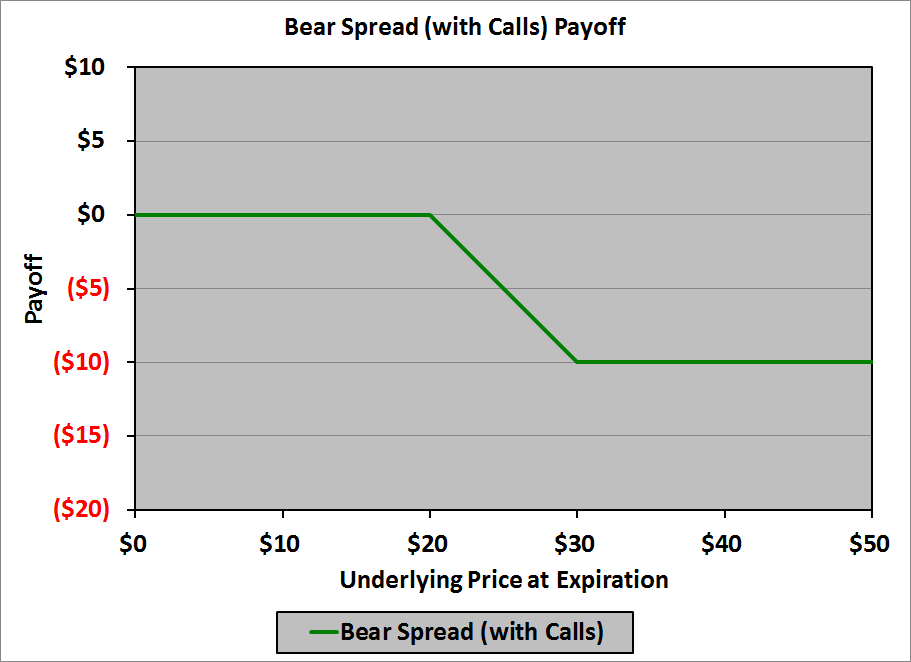In a table:

 Underlying Price at Expiration Payoff $0$0 $20$0 $30 ($10) $50 ($10)

Thus, to create the bear spread with calls, we need:

• A short call with a strike price of $20 • A long call with a strike price of$30

More generally, to create a bear spread with calls, we need:

• A short call with a strike price of X1
• A long call with a strike price of X2

Now let’s create the same bear spread with put options. Our starting point is the same as it was with call options:Here, however, we will start at the right – with an underlying price at expiration of $50 – and work our way left. When we get to$30 we need to add an upward kink to the payoff diagram: a slope of −1. We need an option that has a slope of 0 to the right of $30, and a slope of −1 to the left of$30; a long put option with a strike price of $30 will handle it: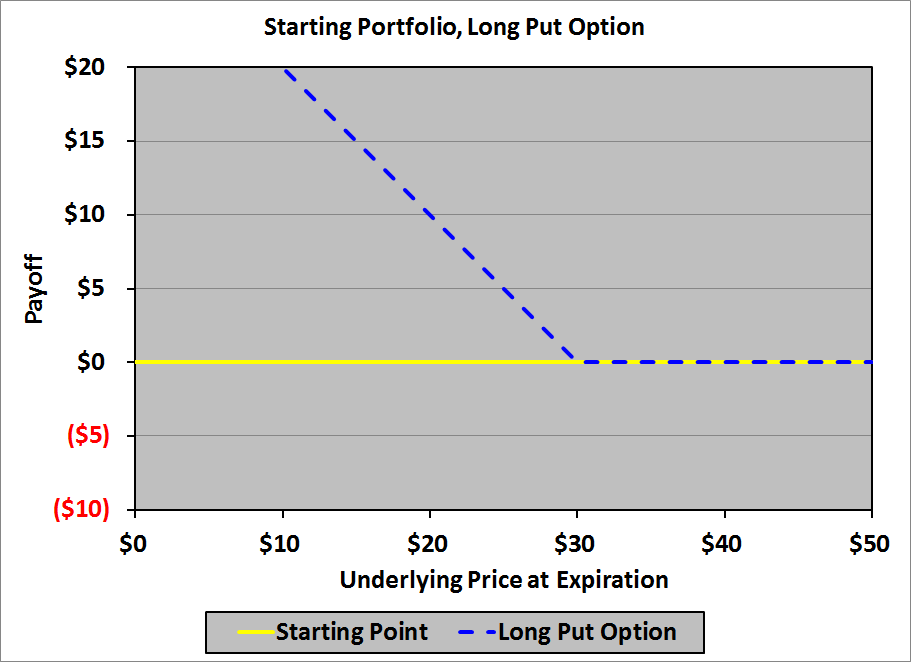This gives us an intermediate payoff diagram that looks like this:Moving farther to the left, when we reach$20, we need to add a downward kink to the diagram: back to horizontal with a slope of zero. To do this, we need an option with a slope of 0 to the right of $20, and a slope of 1 to the left of$20; a short put option with a strike price of $20 does the trick: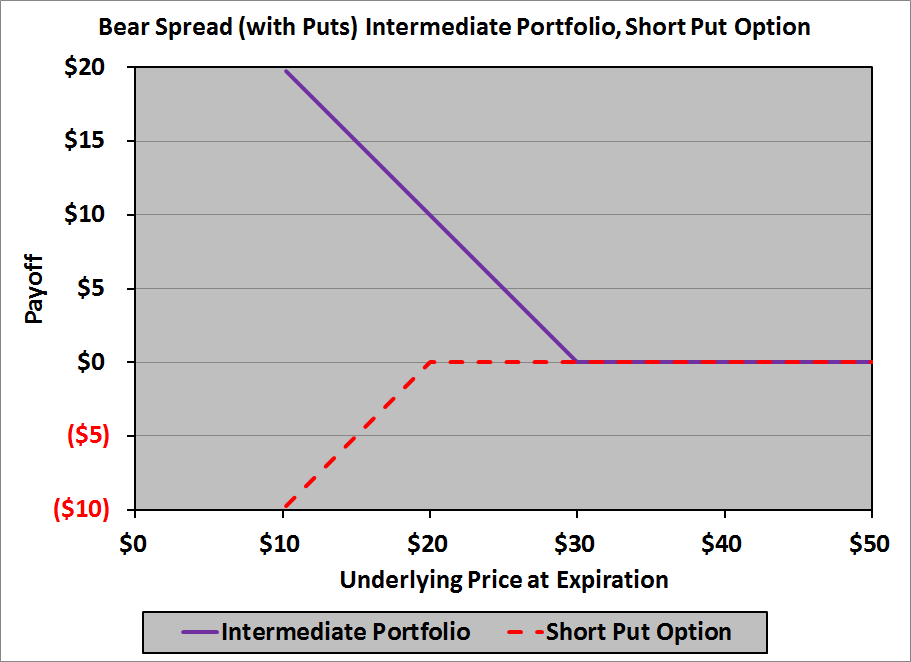This is the last kink, so we have our payoff diagram:In a table:  Underlying Price at Expiration Payoff$0 $10$20 $10$30 $0$50 $0 Thus, to create the bear spread with puts, we need: • A short put with a strike price of$20
• A long put with a strike price of $30 More generally, to create a bear spread with puts, we need: • A short put with a strike price of X1 • A long put with a strike price of X2 Although the payoff diagrams aren’t identical – the payoff with puts is$10 above the payoff with calls for every price of the underlying at expiration – it turns out that the profit diagrams are identical (unless there are mispriced options and, therefore, an arbitrage opportunity) as we’ll see in a moment.

Profit Diagram

To construct the profit diagram, we need to know the cost of each of the options used in constructing the payoff diagram.  For our example, the option costs are derived using the Black-Scholes-Merton pricing model with these parameters:

• Current price of the underlying: $25 • Annual (effective) risk-free interest rate: 2.0% • Time to expiration: 6 months • Annual volatility of underlying’s returns: 30% The option costs are:  Option Type Strike Price Option Cost Call$20 $5.52 Call$30 $0.68 Put$20 $0.32 Put$30 $5.38 The future value (at expiration of the options) of the (net) cost of the option strategy is subtracted from the payoff at each point to get the profit at that point. Bear Call Spread The options needed for the bear spread constructed with calls are a short call with a strike of$20 and a long call with a strike of  $30. The net cost of the bear call spread today is: $\0.68\ -\ \5.52\ =\ -\4.84$ (Note that this is a negative number: the bear call spread actually generates cash today.) The future value (in 6 months, at 2% effective annual interest) of the cost is: $-\4.84\ ×\ 1.02^{6/12}\ =\ -\4.89$ (Note that this is the step you omit on the CFA exam; use −$4.84, not −$4.89.) The profit diagram is:In a table:  Underlying Price at Expiration Profit$0 $4.89$20 $4.89$30 ($5.11)$50 ($5.11) Bear Put Spread The options needed for the bear spread constructed with puts are a short put with a strike of$20 and a long put with a strike of $30. The net cost of the bear call spread today is: $\5.38\ -\ \0.32\ =\ \5.06$ The future value (in 6 months, at 2% effective annual interest) of the cost is: $\5.06\ ×\ 1.02^{6/12}\ =\ \5.11$ (Note that this is the step you omit on the CFA exam; use$5.06, not $5.11.) The profit diagram is: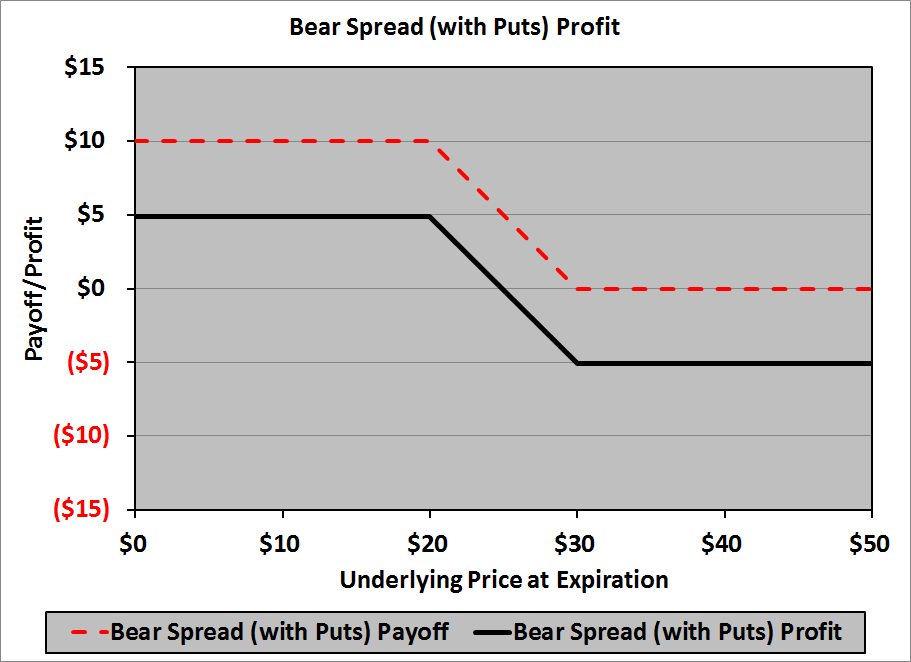In a table:  Underlying Price at Expiration Profit$0 $4.89$20 $4.89$30 ($5.11)$50 ($5.11) Note that the profit of the bear put spread is identical to the profit of the bear call spread no matter the price of the underlying at expiration. This has to happen, of course, if the options are fairly priced today; if the profits were different, there would be an arbitrage opportunity: buy the bear spread with the higher profit and sell the bear spread with the lower profit. Thus, we can talk about the profit of a bear spread, without having to concern ourselves with whether the spread is constructed with calls or puts. Breakeven Price of the Underlying at Expiration Our next concern is the price of the underlying at expiration at which we will break even in the bear spread. Graphically, it looks like this: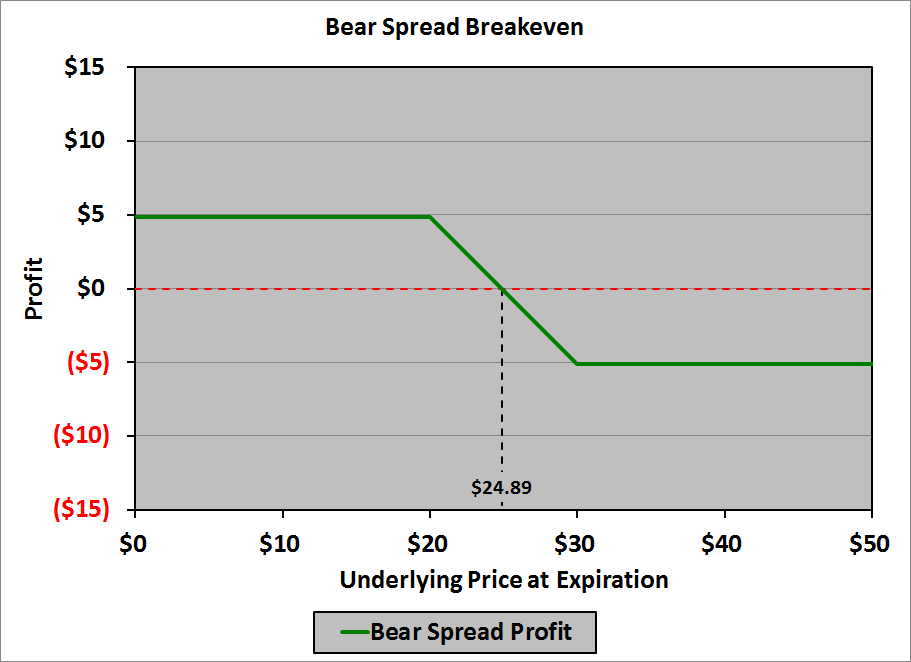In general, the breakeven point will occur at an underlying price between X1 and X2 (between$20 and $30 in our example). Calculating the breakeven price of the underlying at expiration relies on the fact that the middle section of the profit graph has a slope of −1. The profit is$4.89 when the underlying price at expiration is $20. To get to a profit of zero, therefore, we must increase the underlying price by$4.89; thus, the breakeven price of the underlying at expiration is:

$\20.00\ +\ \4.89\ =\ \24.89$

Note, too, that we could start at $30 where the profit is −$5.11; we would need to decrease the underlying price by 5.11 to get a profit of zero; thus, the breakeven price of the underlying at expiration can also be calculated as: $\30.00\ -\ \5.11\ =\ \24.89$ In general, the breakeven price will be: \begin{align}Breakeven\ price\ &=\ X_1\ +\ Profit\ at\ X_1\\ \\ &=\ X_2\ -\ Loss\ at\ X_2 \end{align} Maximum Profit and Maximum Loss Our final concern is the maximum profit achievable or loss incurrable in a bear spread. The easiest way to determine the conditions under which each would occur is to look at the profit diagram: the maximum profit occurs at X1 (20 in our example) and the maximum loss occurs at X2 ($30 in our example). To compute the maximum profit, simply compute the payoff of the constituent options at X1, then subtract the net cost of the options. To compute the maximum loss, simply compute the payoff of the constituent options at X2, then subtract the net cost of the options. In our example, if we built the bear spread using call options:  Underlying Price at Expiration Payoff on$20 Call Payoff on $30 Call Total Payoff Option Cost Profit$20 $0$0 $0$4.89 $4.89$30 ($10)$0 ($10)$4.89 ($5.11) Thus, the maximum profit is$4.89, and the maximum loss is $5.11. If we built the bear spread using put options:  Underlying Price at Expiration Payoff on$20 Put Payoff on $30 Put Total Payoff Option Cost Profit$20 $0$10 $10 ($5.11) $4.89$30 $0$0 $0 ($5.11) ($5.11) Again, the maximum profit is$4.89, and the maximum loss is $5.11. What’s the Point? Why would you choose to employ a bear spread strategy? You would employ a bear spread if you have a mild belief that the price of the underlying is going to decrease, but you’re concerned that the price may increase. The spread protects your downside (and, presumably, your backside), while: • Giving you some upside potential, and • Not costing you a lot. Note that if you had a strong belief that the price of the underlying were going to decrease, you could simply purchase a put option, or create a synthetic short position (buy a put and sell a call with the same strike price, close to today’s spot price). A bear spread suggests a tempered (mild) belief in the depreciation of the underlying, coupled with caution. ## Option Strategies Candidates seem to have a tremendously difficult time with option strategies: how to construct various spreads, how to compute the payoffs on those spreads, how to compute the profits on those spreads, how to compute the breakeven underlying prices on those spreads, and why they would employ a particular spread. I’m here to tell you that it isn’t nearly as hard as most people make it out to be. I kid you not. You can master this stuff, without pharmaceutical assistance. The principle problem, in my experience, is all the max/min junk that you see in the curriculum: it’s confusing as all get-out. Rest assured: you won’t see any max/min functions or formulae in these articles. I’ll show you how to construct the spreads, how to compute the payoffs and profits, and how to determine the break-even points. If you can draw straight lines (technically, line segments and rays), you’re 90% of the way there. (OK, maybe 80%.) The last 10% (20%) is easy. Finally, I’ll explain the reason for each of the spreads: why you would want to use each one. In this series of articles I’ll cover these strategies: The nice thing about these articles is that they have lots of pictures. Preliminaries: Payoffs on Calls and Puts The key to constructing option spreads is a thorough understanding of the payoffs on: • A long call • A long put • A short call • A short put If you do not have these payoffs thoroughly memorized – if you cannot draw them in your sleep, after a long night of partying – you need to practice these with intense determination; they have to be innate . . . second nature. First nature’s even better. Here they are:The horizontal axis in each of these payoff diagrams represents the price of the underlying at the expiration of the optionX is the strike price of each option. The vertical axis is, naturally, the payoff on the option. The horizontal axis crosses the vertical axis at zero. There are some general points about these payoff diagrams that warrant cataloguing: • Long positions (long call, long put) cannot have negative payoffs: the payoff will be zero or positive. (We’re assuming that you’re not an idiot.) • Short positions (short call, short put) cannot have positive payoffs: the payoff will be zero or negative. (We’re also assuming that whoever buys your options is not an idiot.) • Call positions (long call, short call) have nonzero payoffs if and only if the price of the underlying at expiration is higher than the strike price. • Put positions (long put, short put) have nonzero payoffs if and only if the price of the underlying at expiration is lower than the strike price. • Call positions have unlimited positive or negative payoffs: there is no upper limit to the price of the underlying at expiration. (Hence, the arrows in the call payoff diagrams, above.) • Put positions have limited positive or negative payoffs: the price of the underlying cannot fall below zero. (Hence, no arrows in the put payoff diagrams, above.) • For the nonzero portions of these graphs, they rise or fall dollar-for-dollar (or yen-for-yen, or euro-for-euro, or baht-for-baht, or . . . well . . . you get the idea): the slopes are +1 or −1, always. Finally, note that these are payoff diagrams, not profit diagrams. To compute the profit, we need to incorporate the premium paid or received for the option. We’ll handle that at the end of each separate article. We will use these payoff diagrams in our construction of the payoffs of the various spreads listed above. Whenever we have a kink in the payoff diagram for a particular spread, we will have one or more options with strikes at that point. Let’s look at how the various option payoffs affect the shape of an option strategy payoff strategy in general; once we know that, it’s an easy matter to decide what sorts of options we need to achieve a given payoff profile. The effects are perhaps best categorized by considering the slope of a payoff diagram (or a portion of a payoff diagram). The only slopes we’ll see in our payoff diagrams will be 0 (the graph is horizontal), +1 (the graph is going up as we move to the right), and −1 (the graph is going down as we move to the right). Here they are: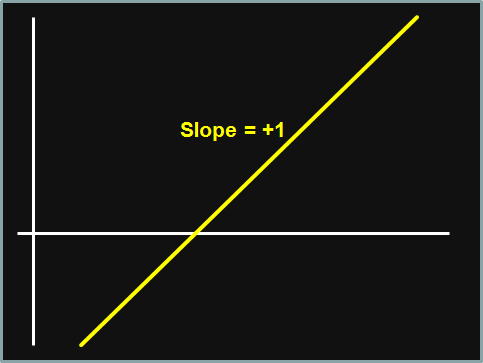Adding a kink to the payoff diagram means changing the slope, which is accomplished by adding an option position. Fortunately, slopes add exactly the way you would expect them to:  Existing Slope Slope Added Resulting Slope −1 + 0 = −1 −1 + +1 = 0 0 + −1 = −1 0 + 0 = 0 0 + +1 = +1 +1 + −1 = 0 +1 + 0 = +1 Graphically, here are some examples of how you can add an option position to create a specific kink in a payoff diagram. In these examples, the yellow graph represents the existing position (without the new option position being added), the (dashed) blue graph represents the payoff of the new option position, and the green graph represents the payoff on the resulting position (with the new option position added). In each case, you should convince yourself that the slope of the green graph at any x (horizontal axis) value is equal to the slope of the yellow graph at that x value plus the slope of the blue graph at that x value. Chromatically, yellow plus blue gives green, so it should be easy to remember what’s what. Example 1Here, the existing payoff diagram (yellow) is constant: horizontal (slope = 0). We add a long call option with a strike price of X (blue): slope = 0 below (to the left of) X, slope = +1 above (to the right of) X. The resulting payoff diagram (green) is horizontal (at the original level) to the left of X; to the right of X the payoff has a slope of +1. If we look at the slopes: to the left of X we have 0 + 0 = 0 (original plus option equals result); to the right of X we have 0 + 1 = +1 (original plus option equals result). In a table, the payoffs might look like this, where the strike on the option is X =$30:

 Underlying Price Original Option New $0.00$10.00 $0.00$10.00 $10.00$10.00 $0.00$10.00 $20.00$10.00 $0.00$10.00 $30.00$10.00 $0.00$10.00 $40.00$10.00 $10.00$20.00 $50.00$10.00 $20.00$30.00 $60.00$10.00 $30.00$40.00

Example 2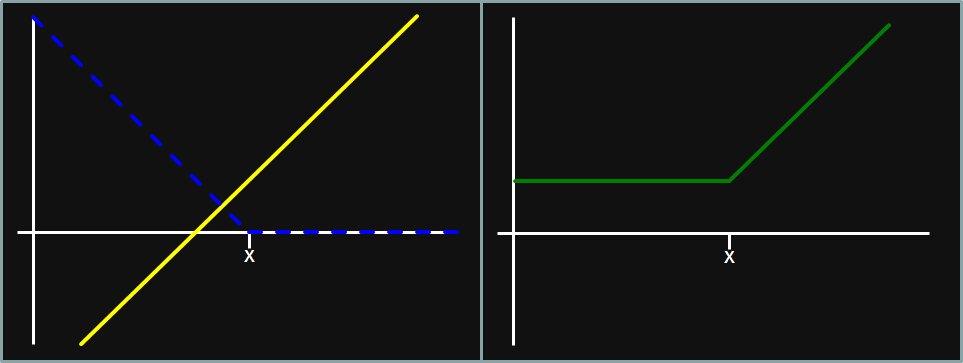Here, the existing payoff diagram (yellow) has a slope of +1.  We add a long put option with a strike price of X (blue): slope = −1 to the left of X, slope = 0 to the right of X.  The resulting payoff diagram (green) is horizontal to the left of X; to the right of X the payoff has a slope of +1.

If we look at the slopes: to the left of X we have (+1) + (−1) = 0 (original plus option equals result); to the right of X we have (+1) + 0 = +1 (original plus option equals result).

In a table, the payoffs might look like this, where the strike on the option is X = $30:  Underlying Price Original Option New$0.00 ($20.00)$30.00 $10.00$10.00 ($10.00)$20.00 $10.00$20.00 $0.00$10.00 $10.00$30.00 $10.00$0.00 $10.00$40.00 $20.00$0.00 $20.00$50.00 $30.00$0.00 $30.00$60.00 $40.00$0.00 $40.00 Example 3Here, the existing payoff diagram (yellow) has a slope of −1. We add a short put option with a strike price of X (blue): slope = +1 to the left of X, slope = 0 to the right of X. The resulting payoff diagram (green) is horizontal to the left of X; to the right of X the payoff has a slope of −1. If we look at the slopes: to the left of X we have (−1) + (+1) = 0 (original plus option equals result); to the right of X we have (−1) + 0 = −1 (original plus option equals result). In a table, the payoffs might look like this, where the strike on the option is X =$30:

 Underlying Price Original Option New $0.00$40.00 ($30.00)$10.00 $10.00$30.00 ($20.00)$10.00 $20.00$20.00 ($10.00)$10.00 $30.00$10.00 $0.00$10.00 $40.00$0.00 $0.00$0.00 $50.00 ($10.00) $0.00 ($10.00) $60.00 ($20.00) $0.00 ($20.00)

Example 4

For a change of pace, let’s look at an existing payoff diagram that already has a kink in it: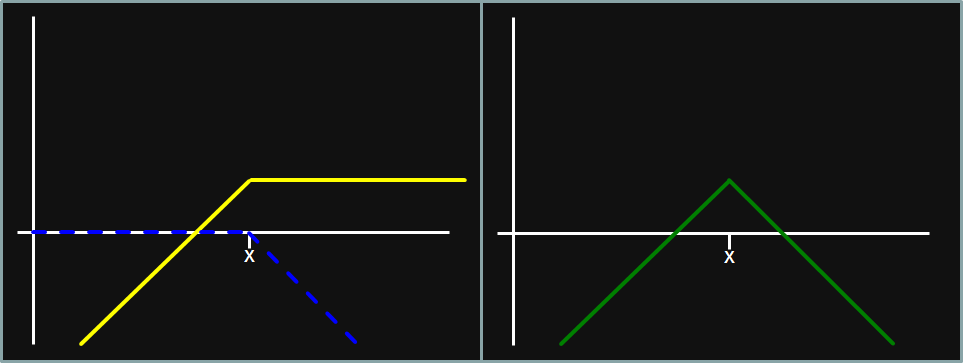Here, the existing payoff diagram (yellow) has a slope of +1 to the left of X, and a slope of 0 to the right of X.  We add a short call option with a strike price of X (blue): slope = 0 to the left of X, slope = −1 to the right of X.  The resulting payoff diagram (green) has a slope of +1 to the left of X; to the right of X the payoff has a slope of −1.

If we look at the slopes: to the left of X we have (+1) + (0) = +1 (original plus option equals result); to the right of X we have 0 + (−1) = −1 (original plus option equals result).

In a table, the payoffs might look like this, where the strike on the option is X = $30:  Underlying Price Original Option New$0.00 ($20.00)$0.00 ($20.00)$10.00 ($10.00)$0.00 ($10.00)$20.00 $0.00$0.00 $0.00$30.00 $10.00$0.00 $10.00$40.00 $10.00 ($10.00) $0.00$50.00 $10.00 ($20.00) ($10.00)$60.00 $10.00 ($30.00) (20.00) At this point you should have the hang of adding kinks to a payoff diagram by adding new option positions. We’ll use this knowledge to build the payoff diagrams for the option strategies listed at the beginning of this article. Our approach to building the payoff diagrams will be methodical: we’ll start at the left and work our way right, adding options for each kink in the payoff diagram. Then, for an exciting change of pace, we’ll build the same diagram by starting at the right and working our way to the left, adding options for each kink along the way. In this way, you’ll see that there is more than one way to build an option strategy payoff diagram, and you’ll gain more insight into how option strategies work in general. It’ll be fun. Let me warn you ahead of time that the one bit of work you’re going to have to put into this exercise – apart from having to learn the shapes of the payoff diagrams for individual options, covered above – is to learn the shapes of the payoff diagrams for each of the option strategies. This is one area where you’ll benefit from drawing the pictures many, many times. Draw the picture and write the name of the strategy. Do this for each strategy. Then throw away the pictures and do it again. Once you’ve done this a few dozen times, you’ll know these diagrams cold. A Word of Caution about Computing the Profit on Option Strategies In the articles on the various option strategies, I explain how to compute the profit on each strategy, which leads to drawing the profit diagram, and forms the basis for determining the breakeven points. In computing the profit, I add a wrinkle that, as far as I can tell, is not discussed explicitly anywhere else: the (net) cost of the option strategy that you subtract from the payoff to get the profit must be the future value of the cost (i.e., the future value at the expiration of the options, when the payoff occurs), not the present value of the cost (i.e., when the strategy is undertaken). The reason that I emphasize that it must be the future value of the (net) cost is that for some of the strategies you get a very different cost if you create the strategy with calls than if you create it with puts (and a correspondingly different payoff for the two constructions). If you (incorrectly) calculate the profit using the present value of the (net) cost, then it appears that the profit using calls is different from the profit using puts, suggesting that there is an arbitrage opportunity. However, if you (correctly) calculate the profit using the future value of the (net) cost, the profit is the same in both cases, as it should be. I make a big point of this because in every textbook on options, in every article you’ll likely ever read on option strategies, and, in particular, in the CFA curriculum, the profit is always shown as being calculated using the present value of the (net) cost. It’s wrong, but there it is. Note well: if you calculate the profit the correct way (using the future value of the (net) cost), you will lose points on the CFA exam; if you calculate the profit the incorrect way (using the present value of the (net) cost), you won’t lose points on the CFA exam. I want you to get the points, so on the exam I want you to do it incorrectly, but I also want you to know that you’re doing it incorrectly. When you get back to work the Monday after the exam, do it correctly. As a help, in each article I’ve added a note every time I compute the future value of the cost, letting you know that that’s the step that you need to omit when you’re taking the Level III CFA exam. ## Cash Flow Matching As an approach to asset-liability management (ALM), cash flow matching has advantages and disadvantages. The primary advantages are that: • It works perfectly. • It is relatively simple to understand. The primary disadvantages are that: • It can be costly (i.e., the interest that you earn on your assets may be low). • It may be difficult to implement in practice. The easiest way to explain cash flow matching is through an example, so here goes: Suppose that you manage a pension fund that has liabilities of €2 million, €4 million, €7 million, and €11 million coming due in 1, 2, 3, and 4 years, respectively. You want to invest in bonds whose cash flows will exactly match the liabilities. The following bonds are available: • 1-year, 2-year, 3-year, and 4-year zero-coupon bonds; the yields (YTMs) are 1%, 2%, 3%, and 4%, respectively • 1-year annual-pay bonds with a coupon rate of 1%, selling at par • 2-year annual-pay bonds with a coupon rate of 2%, selling at par • 3-year annual-pay bonds with a coupon rate of 3%, selling at par • 4-year annual-pay bonds with a coupon rate of 4%, selling at par The easiest way to implement cash-flow matching is with the zero-coupon bonds: buy €2 million par of 1-year bonds, €4 million par of 2-year bonds, €7 million par of 3-year bonds, and €11 million par of 4-year bonds. This will match your cash flows perfectly – the 1-year bonds will mature in 1 year and pay €2 million, the 2-year bonds will mature in 2 years and pay €4 million, and so on, but it will be a costly way to match the cash flows; the outlay today to buy the bonds will be: • €1,980,198 (= €2 million ÷ 1.01) for the 1-year bonds • €3,844,675 (= €4 million ÷ 1.022) for the 2-year bonds • €6,405,992 (= €7 million ÷ 1.033) for the 3-year bonds • €9,402,846 (= €11 million ÷ 1.044) for the 4-year bonds The total cost for these bonds is €21,633,711. The alternative is to use the coupon-paying bonds that have higher yields. To implement cash flow matching with coupon-paying bonds, you need to start with the longest-maturity liability and work back to the shortest. The steps aren’t difficult, but do require that you pay attention to all of the cash flows. The key to understanding this approach is to recall that the final payment on a coupon-paying bond is par plus coupon, so the par value you need is obtained by diving the cash flow that you need by (1 + coupon rate): \begin{align}par\ +\ coupon\ &=\ liability\\ \\ par\ +\ \left(par\ ×\ coupon\ rate\right)\ &=\ liability\\ \\ par\ ×\ \left(1\ +\ coupon\ rate\right)\ &=\ liability\\ \\ par\ &=\ \frac{liability}{1\ +\ coupon\ rate} \end{align} 1. Buy €10,576,923 (= €11,000,000 ÷ 1.04) par of the 4-year, 4% coupon bonds. The final cash flow on these bonds will be par plus the coupon or €10,576,923 + €423,077 = €11,000,000, which will cover the liability in 4 years. Note that these bonds will pay annual coupons of €423,077 one year, two years, and three years from today. 2. As you already have a cash flow of €423,077 at year 3, you need only €6,576,923 (= €7,000,000 − €423,077) more; buy €6,385,362 (= €6,576,923 ÷ 1.03) par of the 3-year, 3% coupon bonds. The final cash flow on these bonds will be par plus the coupon or €6,385,362 + €191,561 = €6,576,923, so the total cash flow at year 3 will be €7,000,000 (= €6,385,362 + €191,561 + €423,077), which will cover the liability in 3 years. Note that these bonds will pay annual coupons of €191,561 one year and two years from today. 3. As you already have cash flows of €423,077 and €191,561 at year 2, you need only €3,385,362 (= €4,000,000 − €423,077 − €191,561) more; buy €3,318,983 (= €3,385,362 ÷ 1.02) par of the 3-year, 2% coupon bonds. The final cash flow on these bonds will be par plus the coupon or €3,318,983 + €66,380 = €3,385,362, so the total cash flow at year 2 will be €4,000,000 (= €3,318,983 + €66,380 + €191,561 + €423,077), which will cover the liability in 2 years. Note that these bonds will pay annual coupons of €66,380 one year from today. 4. As you already have cash flows of €423,077, €191,561, and €66,380 at year 1, you need only €1,318,982 (= €2,000,000 − €423,077 − €191,561 − €66,380) more; buy €1,305,923 (= €1,318,982 ÷ 1.01) par of the 1-year, 1% coupon bonds. The final cash flow on these bonds will be par plus the coupon or €1,305,923 + €13,059 = €1,318,982, so the total cash flow at year 1 will be €2,000,000 (= €1,305,923 + €13,059 + €66,380 + €191,561 + €423,077), which will cover the liability in 1 year. Here are the cash flows in a table, which is probably easier to read:  Cash Flow by Year Bond Year 1 Year 2 Year 3 Year 4 4-year €423,077 €423,077 €423,077 €423,077 + €10,576,923 3-year €191,561 €191,561 €191,561 + €6,385,362 2-year €66,380 €66,380 + €3,318,983 1-year €13,059 + €1,305,923 Total CF €2,000,000 €4,000,000 €7,000,000 €11,000,000 The total cost for this approach is €21,587,191, or €46,520 less than using the zero-coupon bonds. The reason that this approach is less expensive than using only zero-coupon bonds is that the longer maturity bonds have higher yields; so, for example, the €2,000,000 cash flow in year 1 is funded partly by a bond with a 1% yield, and partly by bonds having yields of 2%, 3%, and 4%; using only zero-coupon bonds, the entire €2,000,000 is funded by a bond with a 1% yield. (However, see the Note, below.) The reason that cash flow matching may be difficult to implement in practice is perfectly illustrated by this example: as most euro-denominated bonds have par values of €1,000, it will be impossible to purchase exactly €10,576,923 of 4-year bonds; you would have to decide between purchasing €10,576,000 par (and falling slightly short of your cash flow need) and purchasing €10,577,000 par (and spending too much). The same is true for the par values required at years 3, 2, and 1. Note: in the example given the yields on the zero-coupon bonds and on the coupon-paying bonds of the same maturity are equal. That, of course, shouldn’t happen with an upward-sloping yield curve (lest there be an arbitrage opportunity), but that’s the sort of example that you see in the curriculum. In fact, if the zero-coupon and coupon-paying bonds are priced fairly, there should be no difference in the cost of cash flow matching with zeros and cash flow matching with coupon-paying bonds; once again, if there were a cost difference, there would be an arbitrage opportunity. Sigh. ## Binomial Trees (for Fixed Income) Binomial trees are used in a variety of contexts in finance: In this series of articles, we’ll be concerned with the application of binomial trees to the valuation of bonds. For the other applications, click on the links above. We’ll cover: That’s more than enough. ## Creating a Binomial Interest Rate Tree To create a binomial interest rate tree, you need to start with: 1. A yield curve 2. An interest rate volatility The yield curve can be a par curve, a spot curve, or a forward curve. (If you’re a bit fuzzy on the differences among these curves, look here.) For the remainder of this article, we’ll assume that we’re given a par curve; as we could generate the other curves given any one of them, it doesn’t really matter which one we get. The interest rate volatility will be given as a standard deviation: σ. We’ll see in a moment how we use it to construct the tree. The easiest way to explain how the tree is constructed is by going through an example, so here goes: Suppose that the benchmark (annual pay) par curve is:  Maturity Par Rate 1 2.00% 2 4.00% 3 5.60% 4 6.80% The corresponding spot rates and (1-year) forward rates are:  Maturity Spot Rate Forward Rate 1 2.0000% 2.0000% 2 4.0408% 6.1224% 3 5.7333% 9.2014% 4 7.0587% 11.1355% The (annual) interest rate volatility we’ll use for the tree will be σ = 10%. This is usually implemented as a continuous rate (though there’s no reason that it could not be an effective rate), meaning that we’ll be using a factor of eσ = e10% = 1.1052 to relate low and high interest rates at the same time in the tree. Here’s the tree we’ll be constructing:For each node (i.e., white box), the N simply tells us which node it is: node zero (N0), node low (NL), node high (NH), node low, low (NLL), and so on; the r is the (1-year) forward rate at that node. The keys to creating the binomial interest rate tree are that: • The weights attached to each up leg and each down leg are all 50%. (Many people refer to these as probabilities, which is unfortunate. We’re not asserting anything about the likelihood of forward rates being higher or lower, we’re simply weighting the values we get in the upper leg and the lower leg. You’ll see.) • All of the rates at a given time are related by the assumed volatility of interest rates. • The tree has to give correct prices for (par) bonds. This is known as calibrating the tree. Although it’s trivial, for sake of completeness we’ll start at time t = 0, with r0 at node N0. The 1-year par bond pays a coupon of 2% (20 on a 1,000 par bond), so discounting by r0 has to give us par: \begin{align}\1,000\ &=\ \frac{\$1,000\ +\ \$20}{1\ +\ r_0}\\
\\
\$1,000\left(1\ +\ r_0\right)\ &=\ \$1,020\\
\\
\left(1\ +\ r_0\right)\ &=\ \frac{\$1,020}{\$1,000}\ =\ 1.02\\
\\
r_0\ &=\ 0.02\ =\ 2.00\%
\end{align}

So, not surprisingly, r0 equals the 1-year forward rate starting today, which is the same as the 1-year spot rate, which is the same as the 1-year par rate.  Rest assured, it gets more exciting from here.

Moving to time t = 1, we have two rates to calculate: r1,L at node NL, and r1,H at node NH. We consider r1,L to be one standard deviation down (1σ down) and r1,H to be 1σ up from some sort of mean 1-year forward rate starting 1 year from today (think: up and down from the rate on the forward curve), so the relationship between r1,L and r1,H is that they differ by 2σ:

$r_{1,H}\ =\ e^{2\sigma}r_{1,L}\ =\ e^{20\%}r_{1,L}\ =\ 1.2214r_{1,L}$

The 2-year par bond pays a coupon of 4%, and discounting 50% (the upper weight) along the upper path and 50% (the lower weight) along the lower path (and remembering that we have a coupon payment at time t = 1, and that r0 = 2%) has to give us par:

\begin{align}\$1,000\ &=\ 0.5\left(\frac{\$1,040}{\left(1\ +\ r_{1,H}\right)\left(1.02\right)}\ +\ \frac{$40}{1.02}\right)\\ \\ &+\ 0.5\left(\frac{\$1,040}{\left(1\ +\ r_{1,L}\right)\left(1.02\right)}\ +\ \frac{$40}{1.02}\right)\\ \\ \$1,000\left(1\ +\ r_{1,H}\right)\left(1\ +\ r_{1,L}\right)\left(1.02\right)\ &=\ \$520\left(1\ +\ r_{1,L}\right)\ +\ \$520\left(1\ +\ r_{1,H}\right)\\
\\
&+\ \40\left(1\ +\ r_{1,L}\right)\left(1\ +\ r_{1,H}\right) \end{align} Substituting r1,H = 1.2214r1,L gives: \begin{align}\1,020\left(1\ +\ 1.2214r_{1,L}\right)\left(1\ +\ r_{1,L}\right)\ &=\ \$520\left(1\ +\ r_{1,L}\right)\\ \\ &+\ \$520\left(1\ +\ 1.2214r_{1,L}\right)\\
\\
&+\ \40\left(1\ +\ r_{1,L}\right)\left(1\ +\ 1.2214r_{1,L}\right) \end{align} Multiplying this all out and grouping the terms, we get: $\1,196.97r_{1,L}^2\ +\ \1,021.85r_{1,L}\ -\ 60\ =\ 0$ This is a quadratic equation in r1,L which any high school algebra student could solve using the quadratic formula. (How exciting!) I’ll spare you the details and let you know that the solution that interests us is r1,L = 5.5154%. Thus, r1,H =1.2214(5.5154%) = 6.7365%. (For whatever it’s worth, the other solution to the equation is r1,L = −90.8844%. It’s probably not the one we want to use.) Note that in practice nobody would solve this equation; they use numerical methods to approximate the solution. If you compare these rates to the original forward curve, you’ll see that they look reasonable. The 1-year forward rate starting 1 year from today is 6.1224%. If you compare it to r1,L and r1,H, you get 6.1224% / 5.5153% = 1.1101, and 6.7364% / 6.1224% = 1.1003. Both of these are close to the factor of 1.1052 = e10%, so we should be happy with the results. Moving to time t = 2, we now have three rates to calculate: r2,LL, r2,HL, and r2,HH. They’re related by r2,HL = 1.2214r2,LL and r2,HH = 1.2214r2,LL = 1.4918r2,LL. The 3-year par bond pays a coupon of 5.6%, and if we discount it along all of the paths (4 in total, each with a probability of 25% (= 0.52)), we have to get par. If we follow a similar procedure to the one we used for t = 2, above, we will get a cubic (third-degree) equation for r2,LL. This can be solved using Ferrari‘s formulae (though they were originally discovered by del Ferro and Tartaglia, and published by Cardano), but in practice, the solutions are found numerically. The solution that interests us is r2,LL = 7.4755%, giving r2,HL = 9.1306%, and r2,HH = 11.1521%. Again, if you compare these rates to the original forward curve (the 1-year forward rate starting 2 years from today is 9.2014%), you’ll see that they look reasonable: r2,HL is very close to 1f2: 9.1306% vs. 9.2014%. For time t = 4, we have four rates to calculate, we’re going to get a quartic (fourth-degree) equation for r3,LLL, and we can solve it with Cardano‘s formulae (another of Ferrari‘s brainchildren published by Cardano). Once again, in practice the solutions will be found numerically: we’ll get r3,LLL = 8.1697%, r3,HLL = 9.9785%, r3,HHL = 12.1878%, and r3,HHH = 14.8862%. Comparing these to the forward rate of 11.1355% makes them look reasonable. Beyond time t = 4, we have no choice: we have to proceed numerically. (Abel (building on the work of Ruffini) – and, later, Galois – proved that there is no general algebraic solution to quintic (fifth-degree) and higher-order equations.) The tree now looks like this:Graphically, here’s how the tree looks, compared to the forward curve:Here’s a comparison of the binomial tree with 10% interest rate volatility with one with 20% interest rate volatility: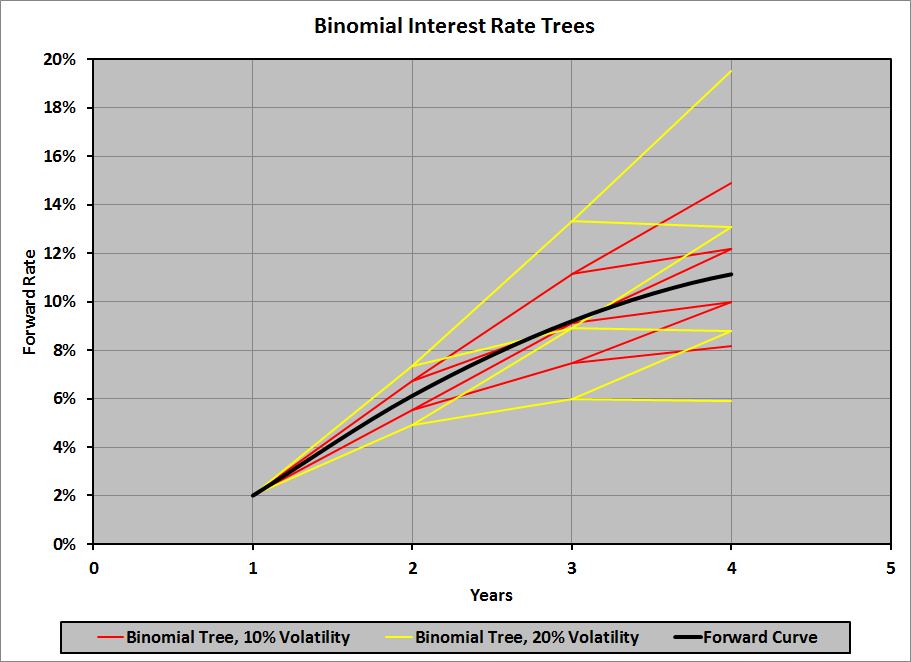To get an idea of how big an impact interest rate volatility can have, here is a 30-year binomial interest rate tree with only 3% annual interest rate volatility; the maximum rate at 30 years is 28.6%:At 5% annual volatility, the maximum rate at 30 years is 51.2%. At 10% annual volatility, the maximum rate at 30 years is 218.1%! ## Key Rate Duration In computing modified (or effective) duration for a portfolio of securities, we change the par interest rate (the yield to maturity) at every maturity by some small amount up and down (±Δy), and determine the percentage price change in the portfolio for 1% change in yield. In essence, we add ±Δy to the entire par curve. (Look here for a refresher on the par curve, and for a refresher on bootstrapping the spot curve from the par curve, which we shall be doing a bit later.) Parallel shifts in the par curve look like this:Modified (or effective) duration is useful for determining how the price of a security or a portfolio of securities will change when the (par) yield curve undergoes a parallel shift, but is less useful (i.e., not useful at all) when the yield curve changes in a manner other than a parallel shift (e.g., flattening or steepening). A more versatile measure of duration is needed in those cases; key rate duration is just such a measure. The idea of a key rate duration (also known as partial duration) is that we don’t add ±Δy to the entire par curve; we add ±Δy only to the YTM at a specific maturity on the par curve, leaving the YTM at all other maturities unchanged; that specific maturity is called the key rate. Thus, we have the: • ½-year key rate duration: ±Δy added to the ½-year YTM, while the YTMs at all other maturities remain unchanged • 2-year key rate duration: ±Δy added to the 2-year YTM • 5-year key rate duration: ±Δy added to the 5-year YTM • 10-year key rate duration: ±Δy added to the 10-year YTM • 20-year key rate duration: ±Δy added to the 20-year YTM • 30-year key rate duration: ±Δy added to the 30-year YTM • And so on Shifts in the par curve to calculate key rate durations look like this: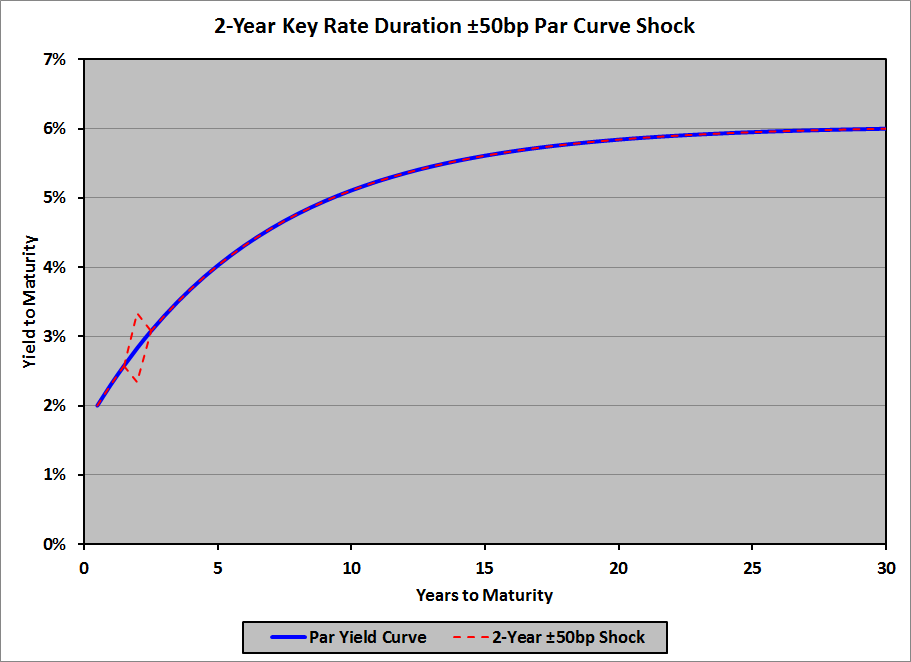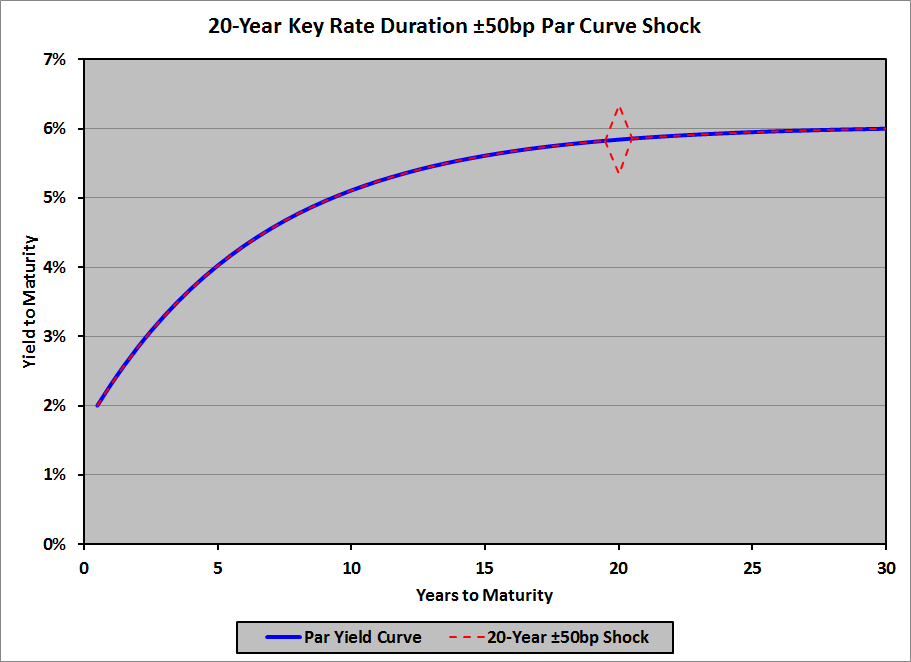Note that key rate durations can be modified (assuming no changes to the cash flows when the key rate changes) or effective (allowing that cash flows might change when the key rate changes). There’s probably no reason to concern yourself about this as far as the exam goes, but in real world applications you might as well assume that key rate durations are effective durations. Once the effect of the key-rate shift is incorporated into the bond price, the duration is computed using the standard (modified or effective) duration formula: $Dur_{key\ rate}\ =\ \frac{P_–\ -\ P_+}{2P_0\Delta y}$ In many respects, key rate durations behave exactly like all other durations (Macaulay duration, modified duration, effective duration, etc.), but in some ways their behavior is unusual. We’ll cover both. Expected Behavior Key Rate Duration of a Portfolio As with Macaulay, modified, and effective duration (see here), any particular key rate duration of a portfolio is the weighted average of that particular key rate duration for each of its constituent bonds, where the weights are based on the market values of the constituent bonds: \begin{align}Dur_{key\ rate_{port}}\ &=\ W_1\ ×\ Dur_{key\ rate_{bond\ 1}}\ +\ W_2\ ×\ Dur_{key\ rate_{bond\ 2}}\\ &+\ \cdots\ +\ W_n\ ×\ Dur_{key\ rate_{bond\ n}}\\ \\ &=\ \sum_{i=1}^n W_i\ ×\ Dur_{key\ rate_{bond\ i}} \end{align} where: • $W_i$: bond i‘s market value ÷ market value of the portfolio So, for example, the 5-year key rate duration of a portfolio of bonds is the weighted average of the 5-year key rate durations of each of the constituent bonds, the 20-year key rate duration of the portfolio is the weighted average of the 20-year key rate durations of each of the constituent bonds, and so on. Relationship of (Modified or Effective) Duration and Key Rate Durations Another characteristic of key rate durations that is expected (or, at least, should be, once you think of it) is that the (modified or effective) duration of a security or a portfolio is the sum of all of its own (respectively, modified or effective) key rate durations: \begin{align}Dur\ &=\ Dur_{key\ rate_1}\ +\ Dur_{key\ rate_2}\ +\ \cdots\ +\ Dur_{key\ rate_k}\ +\cdots\\ \\ &=\ \sum_{i=1}^{\infty}\ Dur_{key\ rate_i} \end{align} Note that this is not an infinite sum: eventually the index i will exceed the maturity of the security or portfolio; after that, all of the key rate durations will be zero (because, as we will see, all of the par rates and all of the spot rates at maturities shorter than the key rate maturity are unchanged). Unusual Behavior Recalling the link between the par curve and the spot curve, we can determine the effect of key rate changes on bonds of various maturities and with various coupons. To illustrate these effects, I’ll use a simple example: a 4% flat yield curve with annual (effective) rates from 1 year to 10 years:  Maturity (Years) Par Yield Spot Yield 1.0 4.0% 4.0% 2.0 4.0% 4.0% 3.0 4.0% 4.0% 4.0 4.0% 4.0% 5.0 4.0% 4.0% 6.0 4.0% 4.0% 7.0 4.0% 4.0% 8.0 4.0% 4.0% 9.0 4.0% 4.0% 10.0 4.0% 4.0% Suppose that we want to compute the 5-year key rate duration of a bond. To do this, we add 50bp to the 5-year par rate (leaving all of the other par rates unchanged), and compute the effect on the spot rates, then subtract 50bp from the 5-year par rate (again, leaving all of the other par rates unchanged), and compute the effect on the spot rates. We’ll then use those modified spot rates to compute $P_-$ and $P_+$, and then use them in the duration formula. Let’s start by adding 50bp to the 5-year par rate, leaving all other par rates unchanged at 4.0%. The first question is: what happens to the 1-year spot yield? The answer is easy: it remains 4.0%. (Recall that the one-period par rate and the one-period spot rate are equal, and that the 1-year par rate hasn’t changed; only the 5-year par rate has changed.) The 2-year spot yield is also easy: it, too, remains 4.0%, because the 2-year par yield is unchanged, and the 1-year spot yield is unchanged. If you want to run through the algebra, it’s: \begin{align}1,000\ &=\ \frac{$40.00}{1.04}\ +\ \frac{$1,040.00}{\left(1\ +\ s_2\right)^2}\\
\\
$1,000\ &=\$38.46\ +\ \frac{$1,040.00}{\left(1\ +\ s_2\right)^2}\\ \\$961.54\ &=\ \frac{$1,040.00}{\left(1\ +\ s_2\right)^2}\\ \\ \left(1\ +\ s_2\right)^2\ &=\ \frac{$1,040.00}{961.54}\ =\ 1.0816\\ \\ 1\ +\ s_2\ &=\ \sqrt{1.0816}\ =\ 1.0400\\ \\ s_2\ &=\ 4.0\% \end{align} The 3-year spot rate and the 4-year spot rate are also easy: they’re both still 4.0%, because their par yields are unchanged, as are all of the spot yields for shorter maturities. I’ll leave the algebra to you. As an exciting (!) change of pace, the 5-year spot rate isn’t 4.0% (recall that we’ve added 50bp to the 5-year par rate). A 5-year bond paying a 4.5% (= 4.0% + 50bp) will therefore sell at par, so: \begin{align}1,000\ &=\ \frac{$45.00}{1\ +\ s_1}\ +\ \frac{$45.00}{\left(1\ +\ s_2\right)^2}\ +\ \frac{$45.00}{\left(1\ +\ s_3\right)^3}\ +\ \frac{$45.00}{\left(1\ +\ s_4\right)^4}\ +\ \frac{$1,045.00}{\left(1\ +\ s_5\right)^5}\\ \\ &=\ \frac{$45.00}{1.04}\ +\ \frac{$45.00}{1.04^2}\ +\ \frac{$45.00}{1.04^3}\ +\ \frac{$45.00}{1.04^4}\ +\ \frac{$1,045.00}{\left(1\ +\ s_5\right)^5}\\
\\
$1,000\ &=\$43.27\ +\ $41.61\ +\$40.00\ +\ $38.47\ +\ \frac{$1,045.00}{\left(1\ +\ s_5\right)^5}\\
\\
$836.65\ &=\ \frac{$1,045.00}{\left(1\ +\ s_5\right)^5}\\
\\
\left(1\ +\ s_5\right)^5\ &=\ \frac{$1,045.00}{$836.65}\ =\ 1.249022\\
\\
1\ +\ s_5\ &=\ 1.249022^{1/5}\ =\ 1.045476\\
\\
s_5\ &=\ 4.5476\%
\end{align}

This result seems reasonable: the YTM is 4.5%, so we’ll get par (1,000) if we discount all of the cash flows at 4.5%. Because we’ve discounted the first four payments at 4% (less than 4.5%), we have to discount the final payment at more than 4.5% to average a 4.5% discount for everything. And because the first four payments are much smaller than the final payment, the difference on the final discount rate should be much smaller than the (50bp) difference on the first four: it’s 4.76bp. Now comes the really interesting part (!!): the 6-year spot rate. Let’s do the algebra (recalling that the 6-year par rate hasn’t changed: it’s still 4.0%): \begin{align}1,000\ &=\ \frac{$40.00}{1.04}\ +\ \frac{$40.00}{1.04^2}\ +\ \frac{$40.00}{1.04^3}\ +\ \frac{$40.00}{1.04^4}\\
\\
&+\ \frac{$40.00}{1.045476^5}\ +\ \frac{$1,040.00}{\left(1\ +\ s_6\right)^6}\\
\\
$1,000\ &=\$38.46\ +\ $36.98\ +\$35.56\ +\ $34.19\ +\$32.03\ +\ \frac{$1,040.00}{\left(1\ +\ s_6\right)^6}\\ \\$822.78\ &=\ \frac{$1,040.00}{\left(1\ +\ s_6\right)^6}\\ \\ \left(1\ +\ s_6\right)^6\ &=\ \frac{$1,040.00}{\$822.78}\ =\ 1.264009\\
\\
1\ +\ s_6\ &=\ 1.264009^{1/6}\ =\ 1.039820\\
\\
s_6\ &=\ 3.9820\%
\end{align}

Again, this result makes sense: the 5-year spot rate has increased, so the only way that the 6-year par rate can remain unchanged is for the 6-year spot rate to decrease.  And, once again, because the final payment is much larger than the first 5 (coupon only) payments, the decrease should be small compared to the increase in the 5-year spot rate: the 5-year spot rate is 54.76bp higher than the 4% YTM, while the 6-year spot rate is 1.80bp lower than the YTM.

Note how the change in the 5-year key rate leads to some somewhat surprising results.  For example, a 6-year zero-coupon bond will have a slightly higher price (because the 6-year spot rate is lower) when the 5-year key rate is increased; in other words, a 6-year zero-coupon bond will have a negative 5-year key rate duration.  (Remember that when interest rates increase, bond prices normally decrease, and that duration is normally positive.  When the price increases with a rate increase, duration must be negative.)

Continuing in this same tedious manner, we get:

 Maturity (Years) Par Yield Spot Yield 1.0 4.0000% 4.0000% 2.0 4.0000% 4.0000% 3.0 4.0000% 4.0000% 4.0 4.0000% 4.0000% 5.0 4.5000% 4.5476% 6.0 4.0000% 3.9820% 7.0 4.0000% 3.9846% 8.0 4.0000% 3.9865% 9.0 4.0000% 3.9880% 10.0 4.0000% 3.9892%

Graphically: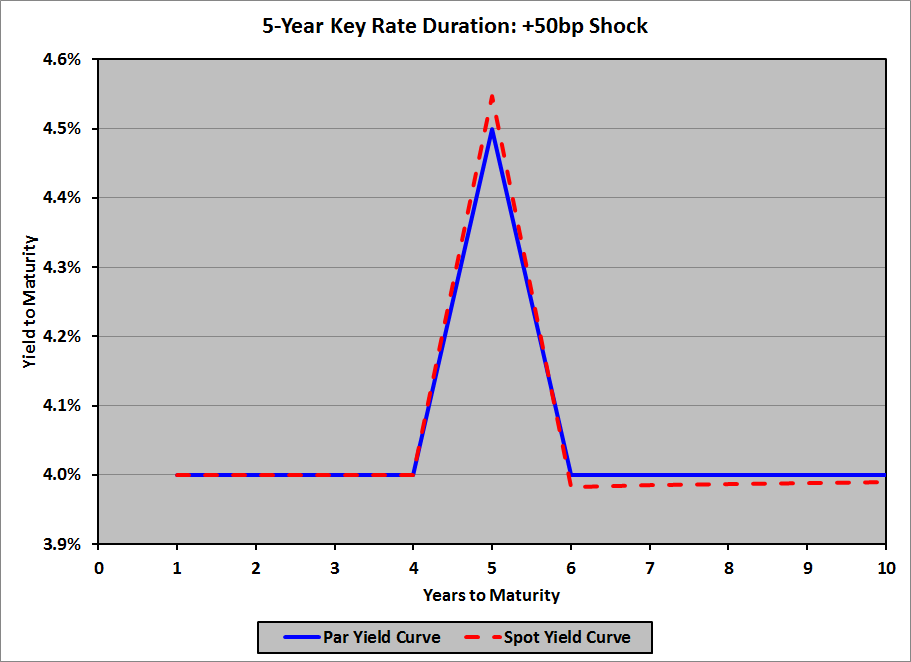When we subtract 50bp from the 5-year par rate, we get:

 Maturity (Years) Par Yield Spot Yield 1.0 4.0000% 4.0000% 2.0 4.0000% 4.0000% 3.0 4.0000% 4.0000% 4.0 4.0000% 4.0000% 5.0 3.5000% 3.4641% 6.0 4.0000% 4.0182% 7.0 4.0000% 4.0156% 8.0 4.0000% 4.0136% 9.0 4.0000% 4.0121% 10.0 4.0000% 4.0109%

Graphically:Key Rate Durations of Various Bonds

Not only does the key rate duration of a bond depend on the bond’s maturity (compared to the maturity of the key rate), it also depends on the bond’s coupon rate compared to its YTM; i.e., it depends on whether the bond is priced at par, at a premium, or at a discount.  Using our 4% flat yield curve, here are the key rate durations for five 5-year, option-free bonds with varying coupon rates, along with the sum of their key rate durations, and their effective durations:

 Key Rate Duration (Years), 5-Year Bond Key Rate Maturity Coupon Rate 0.0% 2.0% 4.0% 6.0% 8.0% 1 Year (0.0385) (0.0174) 0.0000 0.0145 0.0268 2 Years (0.0785) (0.0354) 0.0000 0.0296 0.0547 3 Years (0.1201) (0.0542) 0.0000 0.0453 0.0838 4 Years (0.1633) (0.0737) 0.0000 0.0616 0.1140 5 Years 5.2081 4.7931 4.4519 4.1666 3.9243 6+ Years 0.0000 0.0000 0.0000 0.0000 0.0000 Sum 4.8078 4.6125 4.4519 4.3176 4.2036 DurEff 4.8078 4.6125 4.4519 4.3176 4.2036

So, for example, a 5-year, zero-coupon bond has a 4-year key rate duration of −0.1633 years; if the 4-year par rate increases by 1%, the price of the bond will increase by approximately 0.1633%.  A 5-year, 8% coupon bond has a 3-year key rate duration of 0.0838 years; if the 3-year par rate decreases by 1%, the price of the bond will increase by approximately 0.0838%.

The key ideas to glean from this table are:

• Par bonds have key rate durations of zero years for any key rate maturity shorter than the bond’s maturity
• Discount bonds have negative key rate durations for key rate maturities shorter than the bond’s maturity; in particular, zero-coupon bonds have negative key rate durations for key rate maturities shorter than the bond’s maturity
• Premium bonds have positive key rate durations for key rate maturities shorter than the bond’s maturity
• All bonds have key rate durations of zero years for any key rate maturity longer than the bond’s maturity
• The sum of the key rate durations for all key rate maturities equals the bond’s effective duration

Note that these last two points was already covered under Expected Behavior, above.

Using Key Rate Durations

Key rate durations are used to determine the effect of non-parallel yield curve shifts on a portfolio of bonds.  (In fact, they can also be used to determine the effect of parallel yield curve shifts, although they’re less efficient than simply using the effective duration of each bond.)  For example, suppose that we have this par yield curve:Suppose that we expect the yield curve to flatten: rise 50bp on the short end, fall 50bp on the long end, with a linear transition in between:

 Years Flattening ∆, bp 1.0 50.0 2.0 38.9 3.0 27.8 4.0 16.7 5.0 5.6 6.0 −5.6 7.0 −16.7 8.0 −27.8 9.0 −38.9 10.0 −50.0

The new (par) yield curve will look like this:To determine the effect of this flattening on the value of a fixed income portfolio, we can look at the key-rate durations of each bond in the portfolio, multiply each key rate duration by the yield change (from the table, above) and the bond’s market value, then tot them up.  Note that effective duration (of the portfolio) will not help here, as the shift is not parallel.

Similarly, if we expect the par curve to steepen, or hump, or do anything else other than a parallel shift, key rate durations will allow us to determine the first-order effect on the value of a portfolio of bonds with a range of maturities.

For whatever it’s worth, there’s also the idea of key rate convexities.  I’ve never heard of them – I just came up with the idea all on my own – but for large yield changes it would make sense to consider convexity in addition to duration.  Deep down, I’m a pioneer.

The primary misconception about key rate durations is that they correspond to a change in a single spot rate, instead of a change in a single par rate.  To be clear:

• The key rate duration of a given bond for a given maturity is the ratio of the percentage change in that bond’s price to the change in the par rate at that maturity, when the par rates at all other maturities remain unchanged.
• When the par rate at a given maturity changes, and the par rates at all other maturities remain unchanged:
• The spot rates at maturities less than the given maturity will not change
• The spot rate at the given maturity will change in the same direction as change in the par rate, and by an  amount greater than the change in the par rate
• The spot rates at maturities greater than the given maturity will change in the opposite direction of the change in the par rate, and by an amount (much) less than the change in the par rate (and the change will be smaller at longer maturities)

## Valuing Derivatives

Valuing derivatives – forwards, futures, FRAs, and swaps – is much the same as pricing them.  The value of a derivative is the amount that one party would have to pay the other if the derivative were to expire today; it depends on the price of the underlying today compared to the price at the inception of the derivative.  Therefore, valuing a derivative generally means doing the same sort of calculation you did to price the derivative, then comparing today’s price to the original price.  Finally, bear in mind that the value to one party is the negative of the value to the other party.  I encourage you to practice valuation problems always from the same party’s point of view (e.g., always from long’s point of view in valuing a forward contract); if you have to calculate it from the other party’s point of view on the exam, do it the way you’ve practiced, then change the sign.

Here are links to articles on valuing derivatives:

Also note that the formulae used for valuing derivatives will vary depending on whether the risk-free interest rate is given as an effective rate or a nominal rate; for a refresher on nominal vs. effective interest rates, look here.